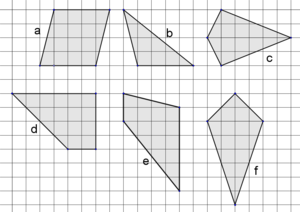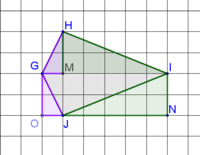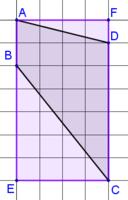##### Engage your students with effective distance learning resources. ACCESS RESOURCES>>

Alignments to Content Standards: 6.G.A.1

Of the polygons shown, which have equal areas? Explain how you know.## IM Commentary

The purpose of this task is for students to use what they know about area to find the areas of special quadrilaterals. Depending on previous instruction, methods may include decomposing the figures into right triangles and rectangles, or drawing a rectangle to encircle the figure and subtracting areas of right triangles that are not part of the original figure. Students may also make use of area formulas that they have previously derived, like $A=bh/2$ for triangles or $A = bh$ for parallelograms. The standards do not expect students to know or use a formula for the area of a trapezoid or area of a kite, though a teacher could use this task as part of a lesson on generating those formulas if so desired.

An open-ended task like this provides a great opportunity for students to explain their reasoning and may lead to student critiques of each other's reasoning MP.3. As students work, teachers should be on the lookout for alternate approaches. Students could be asked to share their approach with the class, or different approaches could be highlighted in something like a gallery walk.

For students who finish quickly, an extension question of slightly higher difficulty might be to ask students to draw additional quadrilaterals with area 24 that are neither rectangles nor parallelograms, or additional polygons with area 24 that include no right angles.

## Solution

Polygons a, d, e, and f each has an area of 16 square units.

(The area of polygon b is 8 square units, and the area of polygon c is 12 square units.)

Depending on previous instruction, methods may include decomposing/composing the figures into right triangles and rectangles [see (1) below], or drawing a rectangle to encircle the figure and subtracting areas of right triangles that are not part of the original figure [see (2) below]. Students may also make use of area formulas that they have previously derived, like A=bh/2 for triangles or A = bh for parallelograms.

(1) The figure below shows polygon c, kite HGJI. Right triangle HGM is relocated to right triangle GJO, and right triangle MIH is relocated to right triangle NJI. This creates rectangle GONI, which has the same area as the original kite, 2 by 6 = 12 square units.(2) The figure below shows polygon e with rectangle AFCE superimposed. If we start with the area of rectangle AFCE (4 by 7 = 28 square units), subtract the areas of triangles AFD (2 square units) and BEC (10 square units), we deduce that the area of polygon e is 28 - 2 - 10 = 16 square units.Instead of calculating the area of each figure, students may instead compare or overlay congruent components of figures. This is a great approach, and discussion could encourage all students to understand several ways to approach the same problem. For example, the figure below shows that polygon c has a smaller area than polygon f.